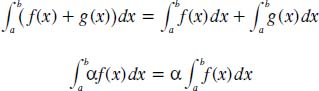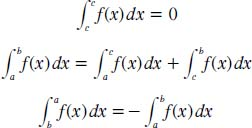NextPrevious

# What are some properties of the definite integral?

There are several useful properties of the definite integral. Theorem one is based on the idea that if f(x) and g(x) are defined and continuous on [a, b], except perhaps at a finite number of points, then the following applies in which alpha is a constant:Theorem two is based on the idea that if f(x) is defined and continuous on [a, b], except at a finite number of points, then the following applies for any arbitrary numbers a and b, and any c ∈ [a, b]:Close

This is a web preview of the "The Handy Math Answer Book" app. Many features only work on your mobile device. If you like what you see, we hope you will consider buying. Get the App